## Problem Set: Arc Length of a Curve and Surface Area

For the following exercises, find the length of the functions over the given interval.

1. $y=5x\text{ from }x=0\text{ to }x=2$

2. $y=-\frac{1}{2}x+25\text{ from }x=1\text{ to }x=4$

3. $x=4y\text{ from }y=-1\text{ to }y=1$

4. Pick an arbitrary linear function $x=g(y)$ over any interval of your choice $({y}_{1},{y}_{2}).$ Determine the length of the function and then prove the length is correct by using geometry.

5. Find the surface area of the volume generated when the curve $y=\sqrt{x}$ revolves around the $x\text{-axis}$ from $(1,1)$ to $(4,2),$ as seen here.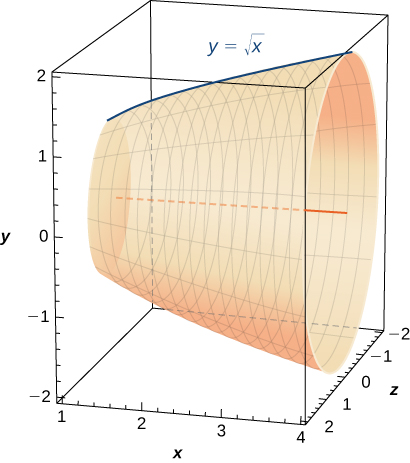6. Find the surface area of the volume generated when the curve $y={x}^{2}$ revolves around the $y\text{-axis}$ from $(1,1)$ to $(3,9).$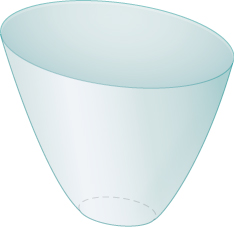For the following exercises (7-16), find the lengths of the functions of $x$ over the given interval. If you cannot evaluate the integral exactly, use technology to approximate it.

7. $y={x}^{3\text{/}2}$ from $(0,0)\text{ to }(1,1)$

8. $y={x}^{2\text{/}3}$ from $(1,1)\text{ to }(8,4)$

9. $y=\frac{1}{3}{({x}^{2}+2)}^{3\text{/}2}$ from $x=0\text{ to }x=1$

10. $y=\frac{1}{3}{({x}^{2}-2)}^{3\text{/}2}$ from $x=2$ to $x=4$

11. [T] $y={e}^{x}$ on $x=0$ to $x=1$

12. $y=\frac{{x}^{3}}{3}+\frac{1}{4x}$ from $x=1\text{ to }x=3$

13. $y=\frac{{x}^{4}}{4}+\frac{1}{8{x}^{2}}$ from $x=1\text{ to }x=2$

14. $y=\frac{2{x}^{3\text{/}2}}{3}-\frac{{x}^{1\text{/}2}}{2}$ from $x=1\text{ to }x=4$

15. $y=\frac{1}{27}{(9{x}^{2}+6)}^{3\text{/}2}$ from $x=0\text{ to }x=2$

16. [T] $y= \sin x$ on $x=0\text{ to }x=\pi$

For the following exercises (17-26), find the lengths of the functions of $y$ over the given interval. If you cannot evaluate the integral exactly, use technology to approximate it.

17. $y=\frac{5-3x}{4}$ from $y=0$ to $y=4$

18. $x=\frac{1}{2}({e}^{y}+{e}^{\text{−}y})$ from $y=-1\text{ to }y=1$

19. $x=5{y}^{3\text{/}2}$ from $y=0$ to $y=1$

20. [T] $x={y}^{2}$ from $y=0$ to $y=1$

21. $x=\sqrt{y}$ from $y=0\text{ to }y=1$

22. $x=\frac{2}{3}{({y}^{2}+1)}^{3\text{/}2}$ from $y=1$ to $y=3$

23. [T] $x= \tan y$ from $y=0$ to $y=\frac{3}{4}$

24. [T] $x={ \cos }^{2}y$ from $y=-\frac{\pi }{2}$ to $y=\frac{\pi }{2}$

25. [T] $x={4}^{y}$ from $y=0\text{ to }y=2$

26. [T] $x=\text{ln}(y)$ on $y=\frac{1}{e}$ to $y=e$

For the following exercises (27-34), find the surface area of the volume generated when the following curves revolve around the $x\text{-axis}.$ If you cannot evaluate the integral exactly, use your calculator to approximate it.

27. $y=\sqrt{x}$ from $x=2$ to $x=6$

28. $y={x}^{3}$ from $x=0$ to $x=1$

29. $y=7x$ from $x=-1\text{ to }x=1$

30. [T] $y=\frac{1}{{x}^{2}}$ from $x=1\text{ to }x=3$

31. $y=\sqrt{4-{x}^{2}}$ from $x=0\text{ to }x=2$

32. $y=\sqrt{4-{x}^{2}}$ from $x=-1\text{ to }x=1$

33. $y=5x$ from $x=1\text{ to }x=5$

34. [T] $y= \tan x$ from $x=-\frac{\pi }{4}\text{ to }x=\frac{\pi }{4}$

For the following exercises (35-42), find the surface area of the volume generated when the following curves revolve around the $y\text{-axis}\text{.}$ If you cannot evaluate the integral exactly, use your calculator to approximate it.

35. $y={x}^{2}$ from $x=0\text{ to }x=2$

36. $y=\frac{1}{2}{x}^{2}+\frac{1}{2}$ from $x=0\text{ to }x=1$

37. $y=x+1$ from $x=0\text{ to }x=3$

38. [T] $y=\frac{1}{x}$ from $x=\frac{1}{2}$ to $x=1$

39. $y=\sqrt{x}$ from $x=1\text{ to }x=27$

40. [T] $y=3{x}^{4}$ from $x=0$ to $x=1$

41. [T] $y=\frac{1}{\sqrt{x}}$ from $x=1$ to $x=3$

42. [T] $y= \cos x$ from $x=0$ to $x=\frac{\pi }{2}$

43. The base of a lamp is constructed by revolving a quarter circle $y=\sqrt{2x-{x}^{2}}$ around the $y\text{-axis}$ from $x=1$ to $x=2,$ as seen here. Create an integral for the surface area of this curve and compute it.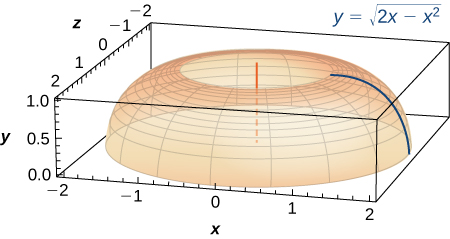44. A light bulb is a sphere with radius $1\text{/}2$ in. with the bottom sliced off to fit exactly onto a cylinder of radius $1\text{/}4$ in. and length $1\text{/}3$ in., as seen here. The sphere is cut off at the bottom to fit exactly onto the cylinder, so the radius of the cut is $1\text{/}4$ in. Find the surface area (not including the top or bottom of the cylinder).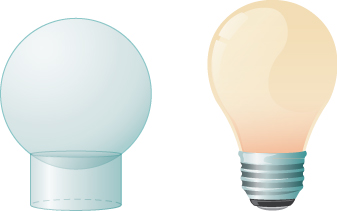45. [T] A lampshade is constructed by rotating $y=1\text{/}x$ around the $x\text{-axis}$ from $y=1$ to $y=2,$ as seen here. Determine how much material you would need to construct this lampshade—that is, the surface area—accurate to four decimal places.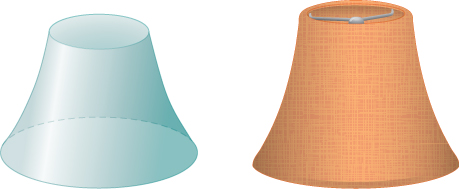46. [T] An anchor drags behind a boat according to the function $y=24{e}^{\text{−}x\text{/}2}-24,$ where $y$ represents the depth beneath the boat and $x$ is the horizontal distance of the anchor from the back of the boat. If the anchor is 23 ft below the boat, how much rope do you have to pull to reach the anchor? Round your answer to three decimal places.

47. [T] You are building a bridge that will span 10 ft. You intend to add decorative rope in the shape of $y=5| \sin ((x\pi )\text{/}5)|,$ where $x$ is the distance in feet from one end of the bridge. Find out how much rope you need to buy, rounded to the nearest foot.

For the following exercises (48-54), find the exact arc length for the following problems over the given interval.

48. $y=\text{ln}( \sin x)$ from $x=\pi \text{/}4$ to $x=(3\pi )\text{/}4.$ (Hint: Recall trigonometric identities.)

49. Draw graphs of $y={x}^{2},$ $y={x}^{6},$ and $y={x}^{10}.$ For $y={x}^{n},$ as $n$ increases, formulate a prediction on the arc length from $(0,0)$ to $(1,1).$ Now, compute the lengths of these three functions and determine whether your prediction is correct.

50. Compare the lengths of the parabola $x={y}^{2}$ and the line $x=by$ from $(0,0)\text{ to }({b}^{2},b)$ as $b$ increases. What do you notice?

51. Solve for the length of $x={y}^{2}$ from $(0,0)\text{ to }(1,1).$ Show that $x=(1\text{/}2){y}^{2}$ from $(0,0)$ to $(2,2)$ is twice as long. Graph both functions and explain why this is so.

52. [T] Which is longer between $(1,1)$ and $(2,1\text{/}2)\text{:}$ the hyperbola $y=1\text{/}x$ or the graph of $x+2y=3?$

54. Explain why the surface area is infinite when $y=1\text{/}x$ is rotated around the $x\text{-axis}$ for $1\le x<\infty ,$ but the volume is finite.